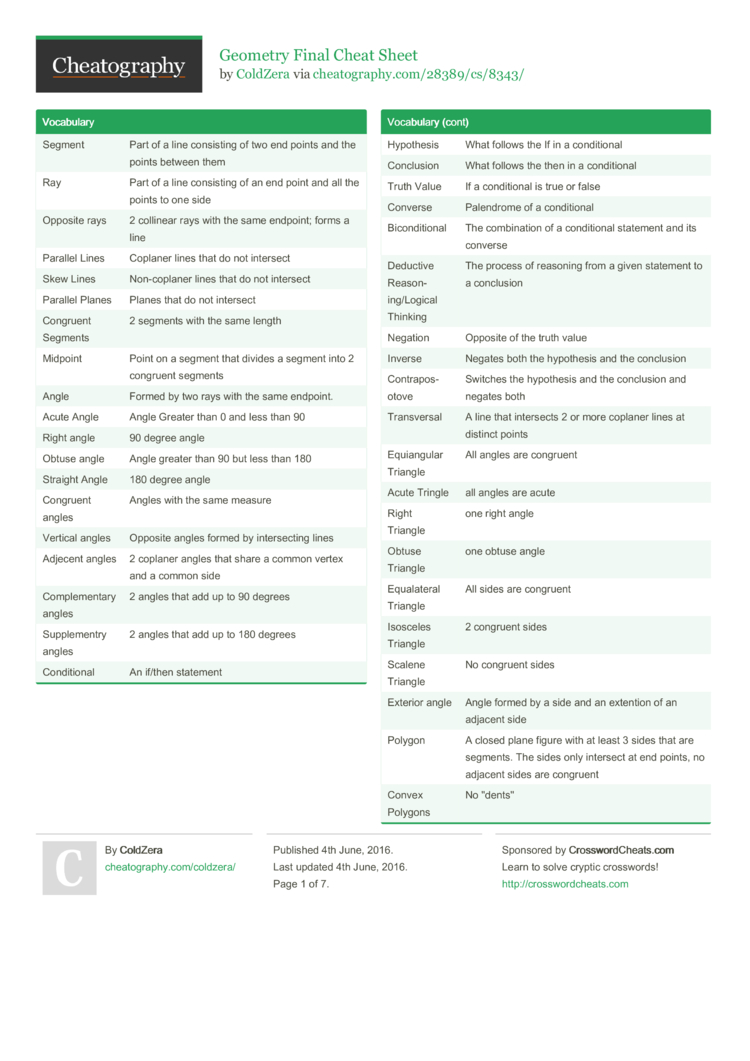# Geometry Final Cheat Sheet by ColdZera

Cheat sheet for my Geometry Final

### All the other crap continued

 Theorem 12-1 If a line is tangent to a circle, then the line is perpen­dicular to the radius drawn to the point of tangency Theorem 12-2 If a line is in the plane of a circle is a radius at its endpoint on the circle, then the line is tangent to the circle Theorem 12-3 The two segments tangent to a circle from a point outside the circle are congruent Perimiter of a Square 4S Area of a Square S2 Perimiter of a Rectan­gle­/Pa­ral­lel­ogram 2B+2H Area of a Rectan­gle­/Pa­ral­lel­ogram BH Circum­ference PiD or 2PiR Area of a Circle PiR2 Perimiter of a Triangle S1+S2+S3 Area of a Triangle .5(b*h) Area of a Trapezoid .5(b1*b2)h Area of a Rhombu­s/Kite .5(d1*d2) Area of Regular Polygons .5AP Arc Addition Postulate The whole is equal to the sum of its parts Arc Length

### Postul­ates, Formulas, etc...6 Pages
//media.cheatography.com/storage/thumb/coldzera_geometry-final.750.jpg

PDF (recommended)

You just saved my grade! Thank you so much!!